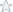Search our library of math homework solutions using Google

<      1      2      3      4

Statistics Questions on Normal Distribution

Topic: Normal Distribution
Details: 1. Find the probability for the standard normal random variable z P(-3.08=z=3.08) 2. Find the area under the standard normal probability distribution between the following pairs of Z-scores z=0 and z=2.00 z=0 and z=1.00 z=0 and z=3.00 z=0 and...
Tags: Statistics Homework Solutions
Rating:5.00 of 5 Stars based on 5 review(s)  [Download Solutions]

Statistics Questions and Answers

Topic: Hypothesis Testing
Details: 1. With a mean of 40 and a standard deviation of 4, find the Z score for a score of 45. 2. What proportion of the area under the standard normal curve would you expect to be between z = 1.2 and z = 0.6 3. What proportion of the area under the stand...
Tags: Statistics Homework Solutions
Rating:3.40 of 5 Stars based on 22 review(s)  [Download Solutions]

Math 115 Project 3 for Chapter 8

Topic: Statistics
Details: Math 115 – Project 3 Please do the following on a separate sheet of paper, in order, and submit in Blackboard inside the Project 3 assignment, as one pdf or one doc file. If you cannot make one file, you must submit your files in a zip folder, still in ...
Tags: Statistics Homework Solutions
Rating:5.00 of 5 Stars based on 1 review(s)  [Download Solutions]

Math 138 - A Comprehensive Statistical Project - Using StatCrunch

Topic: Math 138 Project on Wage Data
Details: A Comprehensive Statistical Project (Before CH 25) Using StatCrunch Total = 45 points Instructions for completing Project Answers to the project must: 1. Be submitted online, using the Assignment or Module Tools by the due date. 2. Include at leas...
Tags: boxplots, statcrunch, advanced statistics
Rating:4.69 of 5 Stars based on 3 review(s)  [Download Solutions]